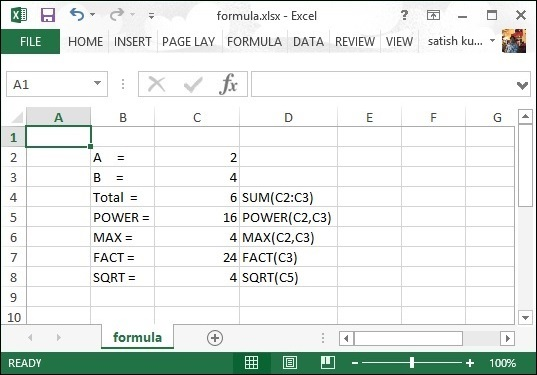# Apache POI - Formula

This chapter takes you through the process of applying different formulas on cells using Java programming. The basic purpose of Excel application is to maintain numerical data by applying formulas on it.

In a formula, we pass dynamic values or locations of the values in the Excel sheet. On executing this formula, you get the desired result. The following table lists a few basic formulas that are frequently used in Excel.

Operation Syntax
Adding multiple numbers = SUM(Loc1:Locn) or = SUM(n1,n2,)
Count = COUNT(Loc1:Locn) or = COUNT(n1,n2,)
Power of two numbers = POWER(Loc1,Loc2) or = POWER(number, power)
Max of multiple numbers = MAX(Loc1:Locn) or = MAX(n1,n2,)
Product = PRODUCT(Loc1:Locn) or = PRODUCT(n1,n2,)
Factorial = FACT(Locn) or = FACT(number)
Absolute number = ABS(Locn) or = ABS(number)
Today date =TODAY()
Converts lowercase = LOWER(Locn) or = LOWER(text)
Square root = SQRT(locn) or = SQRT(number)

The following code is used to add formulas to a cell and execute it.

```import java.io.File;
import java.io.FileOutputStream;
import org.apache.poi.xssf.usermodel.XSSFCell;
import org.apache.poi.xssf.usermodel.XSSFRow;
import org.apache.poi.xssf.usermodel.XSSFSheet;
import org.apache.poi.xssf.usermodel.XSSFWorkbook;

public class Formula {
public static void main(String[] args)throws Exception {
XSSFWorkbook workbook = new XSSFWorkbook();
XSSFCell cell = row.createCell(1);

cell.setCellValue("A = ");
cell = row.createCell(2);
cell.setCellValue(2);
cell = row.createCell(1);
cell.setCellValue("B = ");
cell = row.createCell(2);
cell.setCellValue(4);
cell = row.createCell(1);
cell.setCellValue("Total = ");
cell = row.createCell(2);

// Create SUM formula
cell.setCellFormula("SUM(C2:C3)");
cell = row.createCell(3);
cell.setCellValue("SUM(C2:C3)");
cell = row.createCell(1);
cell.setCellValue("POWER =");
cell=row.createCell(2);

// Create POWER formula
cell.setCellFormula("POWER(C2,C3)");
cell = row.createCell(3);
cell.setCellValue("POWER(C2,C3)");
cell = row.createCell(1);
cell.setCellValue("MAX = ");
cell = row.createCell(2);

// Create MAX formula
cell.setCellFormula("MAX(C2,C3)");
cell = row.createCell(3);
cell.setCellValue("MAX(C2,C3)");
cell = row.createCell(1);
cell.setCellValue("FACT = ");
cell = row.createCell(2);

// Create FACT formula
cell.setCellFormula("FACT(C3)");
cell = row.createCell(3);
cell.setCellValue("FACT(C3)");
cell = row.createCell(1);
cell.setCellValue("SQRT = ");
cell = row.createCell(2);

// Create SQRT formula
cell.setCellFormula("SQRT(C5)");
cell = row.createCell(3);
cell.setCellValue("SQRT(C5)");
workbook.getCreationHelper().createFormulaEvaluator().evaluateAll();
FileOutputStream out = new FileOutputStream(new File("formula.xlsx"));
workbook.write(out);
out.close();
System.out.println("fromula.xlsx written successfully");
}
}
```

Save the above code as Formula.java and then compile and execute it from the command prompt as follows −

```\$javac Formula.java
\$java Formula
```

It will generate an Excel file named formula.xlsx in your current directory and display the following output on the command prompt.

```fromula.xlsx written successfully
```

The formula.xlsx file looks as follows −